## Multinomial Distribution

Let a set of random variates,, ...,have a probability function(1)

whereare Positive Integers,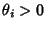, and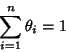(2)(3)

Then the joint distribution of, ...,is a multinomial distribution and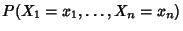is given by the corresponding coefficient of the Multinomial Series(4)

The Mean and Variance ofare(5)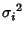(6)

The Covariance ofand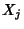is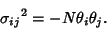(7)